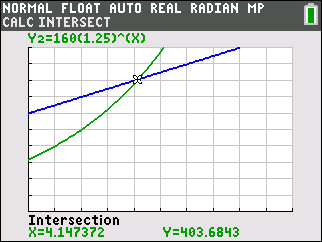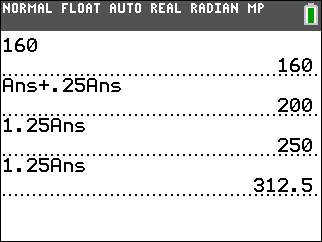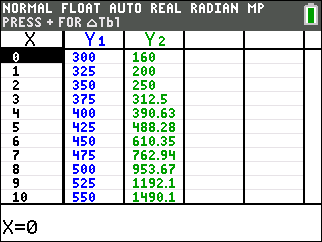# Activities

••• ##### Subject Area

• Math: Middle Grades Math: Expressions and Equations
• Math: Algebra I: Equations
• Math: Algebra I: Exponential Functions

• ##### Author6-8
9-12

45 Minutes

• ##### Device
• TI-84 Plus
• TI-84 Plus Silver Edition
•TI-84 Plus C Silver Edition
•TI-84 Plus CE

## Guppies and Frogs#### Activity Overview

In this activity, students will create equations with two or more variables to represent relationships between quantities. They will also graph equations on coordinate axes with labels and scales.

#### Key Steps

•Students will write functions that describe a linear and exponential relationship between two quantities.

•Students will interpret and compare the average rate of change of a function with the average percent change of a function.

•Students will analyze the equations and graphs of linear and exponential models to determine properties of the models.